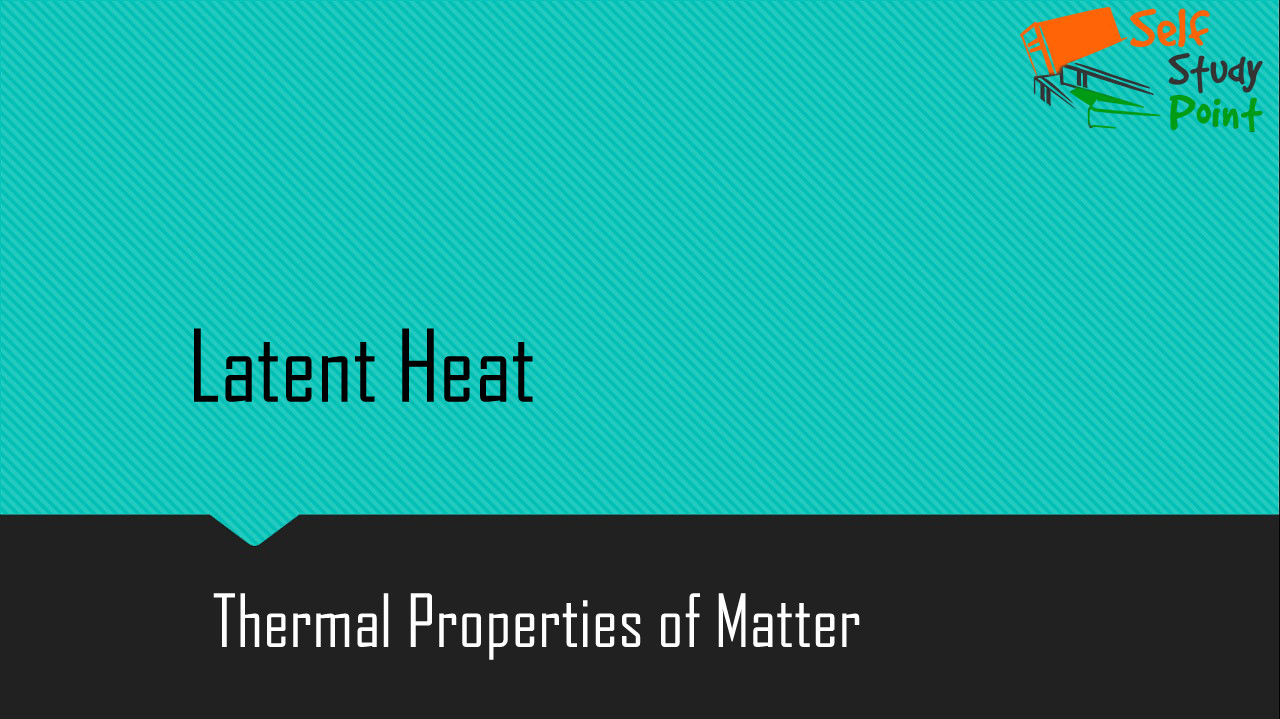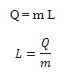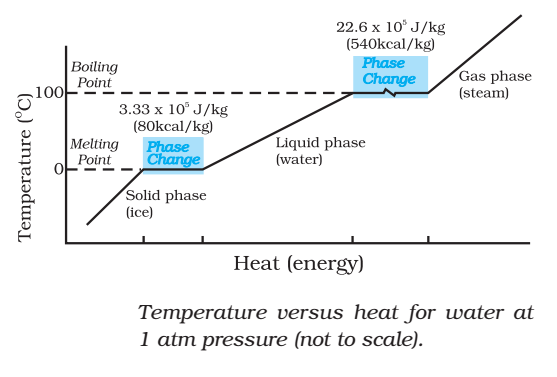The heat required during a change of state depends upon the heat of transformation and the mass of the substance undergoing a change of state. Thus, if mass m of a substance undergoes a change from one state to the other, then the quantity of heat required is given bywhere L is known as latent heat and is a characteristic of the substance.

Its SI unit is J kg-1. The value of L also depends on the pressure.## Latent heat of Fusion:

The latent heat of fusion (Lf) is the heat per unit mass required to change a substance from solid into liquid at the same temperature and pressure.

Lf = 3.3 × 105 J kg-1

## Latent heat of Vaporization:

The latent heat of vaporization (LV) is the heat per unit mass required to change a substance from liquid to the vapor state without the change in the temperature and pressure.

LV = 22.6 × 105 J

Click on a star to rate it!

Average rating / 5. Vote count:

No votes so far! Be the first to rate this post.